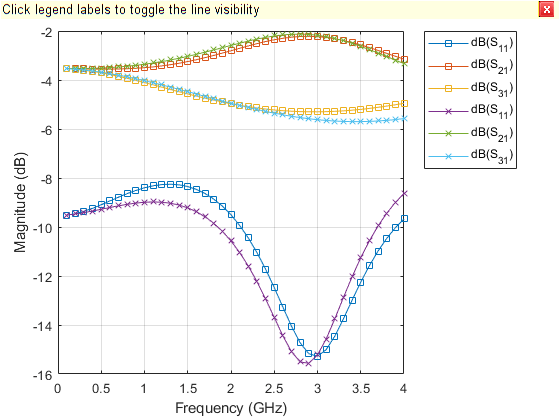# traceTee

Create tee trace

Since R2021b

## Description

Use the `traceTee` object to create a tee trace on the X-Y plane.

Note

This shape object supports behavioral modeling. For more information, see Behavioral Models.

## Creation

### Syntax

``trace = traceTee``
``trace = traceTee(Name=Value)``

### Description

example

````trace = traceTee` creates a tee trace with default properties on the X-Y plane.```
````trace = traceTee(Name=Value)` sets Properties using one or more name-value arguments. For example, `traceTee(ReferencePoint=[1 1])` creates a tee trace with the reference point `[1 1]`. Properties not specified retain their default values.```

## Properties

expand all

Name of the tee trace, specified as a character vector or a string scalar.

Example: `trace = traceTee(Name="traceTeeShape")`

Data Types: `char` | `string`

Reference point of the tee trace in meters, specified as a two-element vector of nonnegative elements.

Example: `trace = traceTee(ReferencePoint=[1 1])`

Data Types: `double`

Length of the horizontal and vertical lines in meters, specified as a two-element vector of positive elements.

Example: `trace = traceTee(Length=[0.0300 0.0200])`

Data Types: `double`

Width of the horizontal and vertical lines in meters, specified as a two-element vector of positive elements.

Example: `trace = traceTee(Width=[0.0060 0.0060])`

Data Types: `double`

Offset along the X-axis in meters, specified as a nonnegative scalar.

Example: `trace = traceTee(Offset=0.0005)`

Data Types: `double`

## Object Functions

 `add` Boolean unite operation on two RF PCB shapes `subtract` Boolean subtraction operation on two RF PCB shapes `intersect` Boolean intersection operation on two RF PCB shapes `plus` Shape1 + Shape2 for RF PCB shapes `minus` Shape1 - Shape2 for RF PCB shapes `and` Shape1 & Shape2 for RF PCB shapes `area` Calculate area of RF PCB shape in square meters `rotate` Rotate RF PCB shape about defined axis `rotateX` Rotate RF PCB shape about x-axis `rotateY` Rotate RF PCB shape about y-axis and angle `rotateZ` Rotate RF PCB shape about z-axis `translate` Move RF PCB shape to new location `scale` Change size of RF PCB shape by fixed amount

## Examples

collapse all

Create a tee trace with default properties.

`trace = traceTee`
```trace = traceTee with properties: Name: 'mytraceTeeShape' ReferencePoint: [0 0] Length: [0.0200 0.0100] Width: [0.0050 0.0050] Offset: 0 ```

View the trace.

`show(trace)`Design a microstrip transmission line at 3 GHz for FR4 substrate.

`m = design(microstripLine('Substrate',dielectric('FR4')),3e9);`

Create a microstrip T-junction.

```layer2d = traceTee('Length',[m.Length m.Length/4],... "Width",[m.Width m.Width/2]);```

Convert the T-junction trace to a 3-D component.

```robj = pcbComponent(layer2d); robj.BoardThickness = m.Substrate.Thickness; robj.Layers{2} = m.Substrate; show(robj)```Define frequency points to calculate the s-parameters.

`freq = (1:40)*100e6;`

Calculate the s-parameters of the T-junction trace using the behavioral model.

`Sckt = sparameters(robj,freq,75,'Behavioral',true);`
```Warning: Behavioral model is valid only when Z0 of main line is 50 ohms and for EpsilonR of 9.9. ```

Calculate the s-parameters of the T-junction trace using the electromagnetic solver.

`Sem = sparameters(robj,freq,75)`
```Sem = sparameters: S-parameters object NumPorts: 3 Frequencies: [40x1 double] Parameters: [3x3x40 double] Impedance: 75 rfparam(obj,i,j) returns S-parameter Sij ```

Plot the s-parameter data using the `rfplot` function.

```rfplot(Sckt,1:3,1,'db','-s') hold on rfplot(Sem,1:3,1,'db','-x')```References:

1. Ramesh Garg & I. J. Bahl (1978) Microstrip discontinuities, International Journal of Electronics, 45:1, 81-87, DOI: 10.1080/00207217808900883

2. Wadell, Brian C. Transmission Line Design Handbook. The Artech House Microwave Library. Boston: Artech House, 1991.

## Version History

Introduced in R2021b